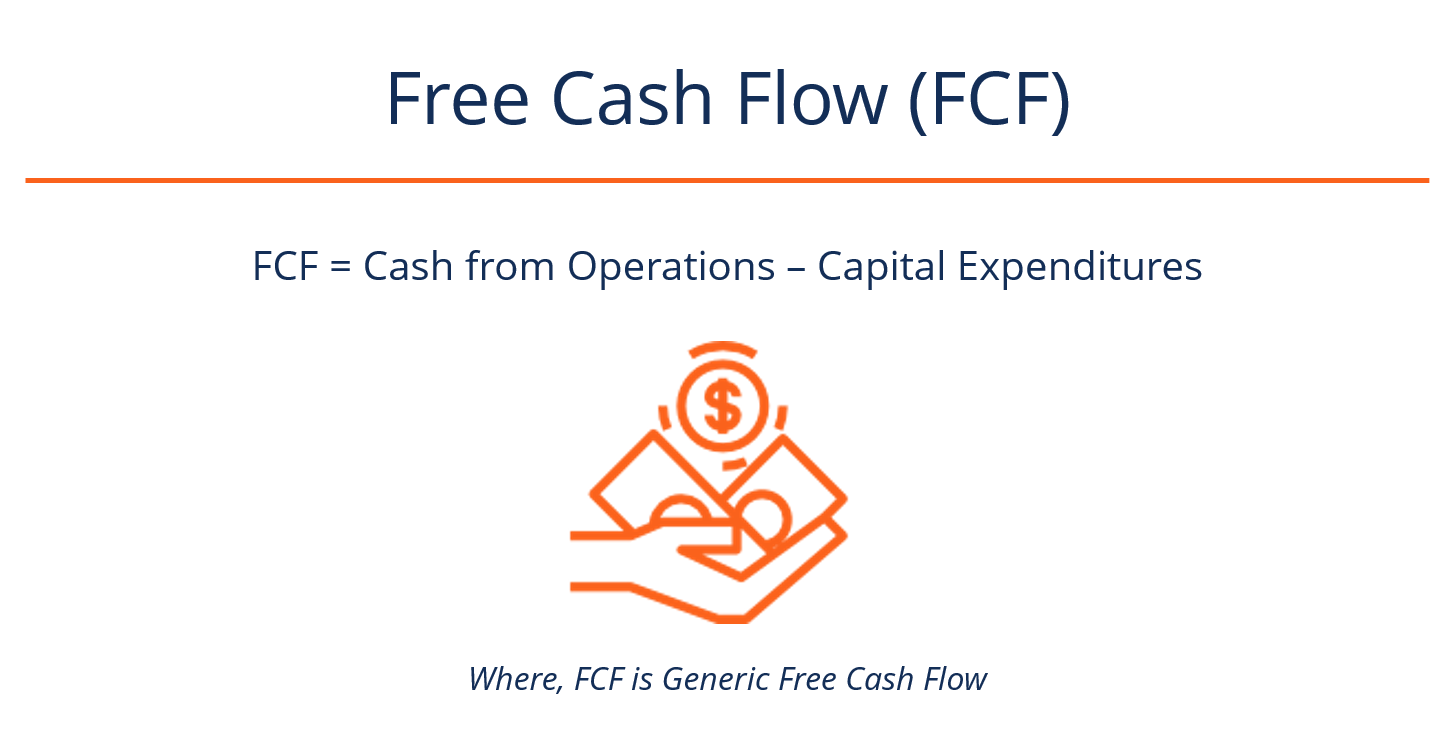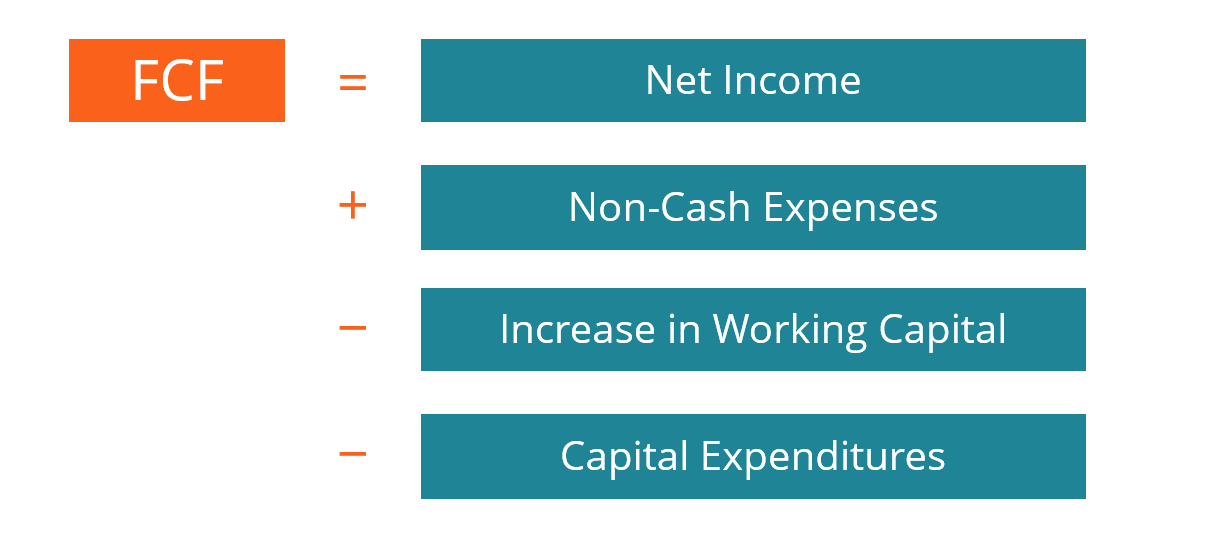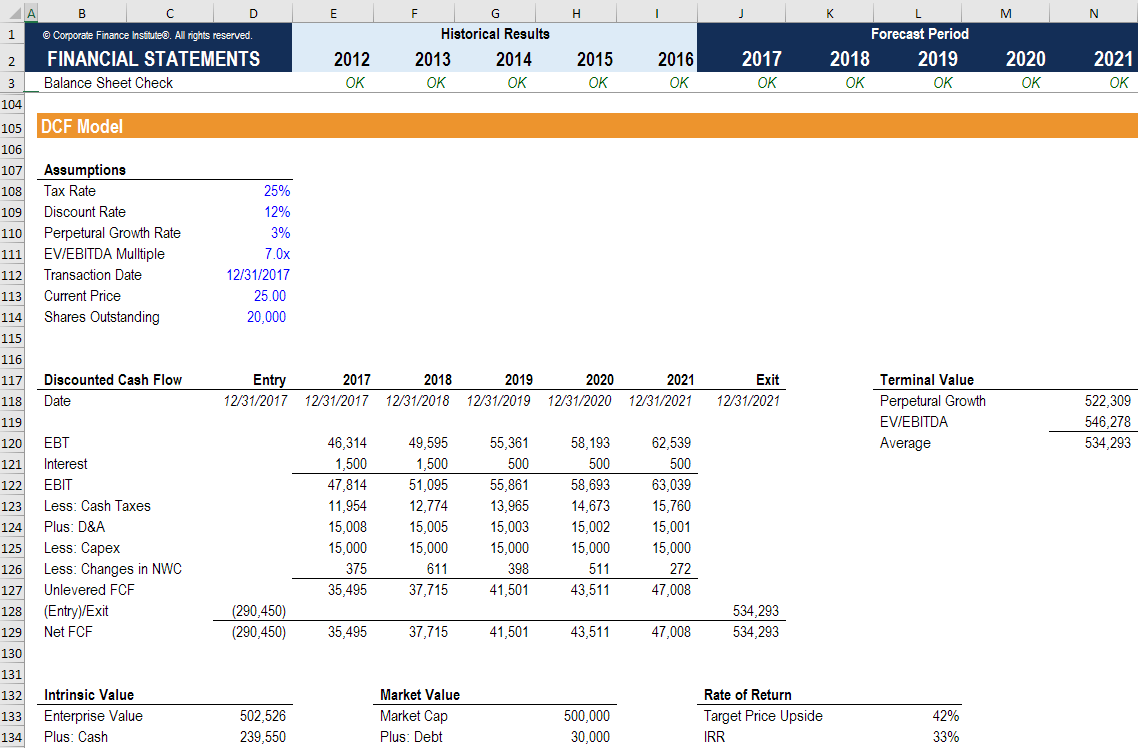# Free Cash Flow (FCF) Formula

The Generic Free Cash Flow Formula

## What is the Free Cash Flow (FCF) Formula?

The generic Free Cash Flow FCF Formula is equal to Cash from Operations minus Capital Expenditures.  FCF represents the amount of cash generated by a business, after accounting for reinvestment in non-current capital assets by the company. This figure is also sometimes compared to Free Cash Flow to Equity or Free Cash Flow to the Firm (see a comparison of cash flow types).Formula:

FCF = Cash from Operations – CapEx

### How to Derive the Free Cash Flow Formula

If you don’t have the cash flow statement handy to find Cash From Operations and Capital Expenditures, you can derive it from the Income statement and balance sheet. Below we will walk through each of the steps required to derive the FCF Formula from the very beginning.#### Step #1 Cash From Operations and Net Income

Cash From Operations is net income plus any non-cash expenses, adjusted for changes in non-cash working capital (accounts receivable, inventory, accounts payable, etc).

Thus, the formula for Cash From Operations (CFO) is:

CFO = Net Income + non-cash expenses – increase in non-cash net working capital

#### Step #2 Non-Cash Expenses

We can further break down non-cash expenses into simply the sum of all items listed on the income statement that do not affect cash.

The most common items that do not affect cash are depreciation and amortization, stock-based compensation, impairment charges, and gains/losses on investments.

Thus, the formula for non-cash adjustments is:

Adjustments = depreciation + amortization + stock-based compensation + impairment charges + gains/losses on investments

#### Step #3 Changes in Non-Cash Net Working Capital

Calculating the changes in non-cash net working capital is typically the most complicated step in deriving the FCF Formula, especially if the company has a complex balance sheet.

The most common items that impact the formula (on a simple balance sheet) are accounts receivable, inventory, and accounts payable.

Thus, the formula for changes in non-cash working capital is:

Changes  =  (2017 AR – 2016 AR)  +  (2017 Inventory – 2016 Inventory)  –  (2017 AP – 2016 AP)

Where,

AR = accounts receivable

AP = accounts payable

2017 = current period

2016 = prior period

#### Step #4 Capital Expenditures

It is possible to derive capital expenditures (CapEx) for a company without the cash flow statement.  To do this, we can use the following formula with line items from the balance sheet and income statement.

Thus, the formula for capital expenditures is:

CapEx = 2017 PP&E – 2016 PP&E + Depreciation & Amortization

#### Step #5 Combining the components of the FCF Formula

We can combine the above four steps into one long FCF formula.

The Full FCF Formula is equal to:

FCF = Net Income  +  [depreciation + amortization + stock-based compensation + impairment charges + gains/losses on investments]  –  [(2017 AR – 2016 AR)  +  (2017 Inventory – 2016 Inventory)  –  (2017 AP – 2016 AP)]  –  [2017 PP&E – 2016 PP&E + Depreciation & Amortization]

or

FCF = Net Income + Non-Cash Expenses – Incrase in Working Capital – Capital Expenditures

In practical terms, it would not make sense to calculate FCF all in one formula.  Instead, it would usually be done as several separate calculations, as we showed in the first 4 steps of the derivation.

The simplified formula is:

FCF = Cash from Operations – CapEx

### Levered and Unlevered Free Cash Flow

When corporate finance professionals refer to Free Cash Flow, they also may be referring to Unlevered Free Cash Flow, (Free Cash Flow to the Firm), or Levered Free Cash Flow (Free Cash Flow to Equity).

One of the main differences between generic Free Cash Flow and Unlevered Free Cash Flow is that regular FCF includes the company’s interest expense, whereas the unlevered version backs out the interest expense and makes an estimate of what taxes would be without the interest expense.

To learn more, see our guide on FCF vs Unlevered FCF vs Levered FCF.

### Video Explanation of Free Cash Flow (Various Types)

Below is a video explanation of various types of cash flow including, EBITDA, CF, FCF, FCFE, and FCFF. Check out the video to learn what each of them is!

Hopefully, this free YouTube video has helped shed some light on the various types of cash flow, how to calculate them, and what they mean. To make sure you have a thorough understanding of each type, please read CFI’s Cash Flow Comparision Guide.

### The FCF Formula in Financial Modeling and Valuation

When it comes to financial modeling and performing company valuations in Excel, most analysts use unlevered FCF.  They will typically create a separate schedule in the model where they break down the calculation into simple steps and all components together.

Below is an example of the unlevered FCF calculation from a real financial model.### More Relevant Resources

We hope this has been a helpful guide to understanding the FCF formula, how to derive it, and how to calculate FCF yourself.

CFI is the official provider of the global Financial Modeling & Valuation Analyst (FMVA)® certification program, designed to help anyone become a world-class financial analyst. To keep advancing your career, the additional resources below will be useful:

• Cash flow guide
• Financial modeling best practices